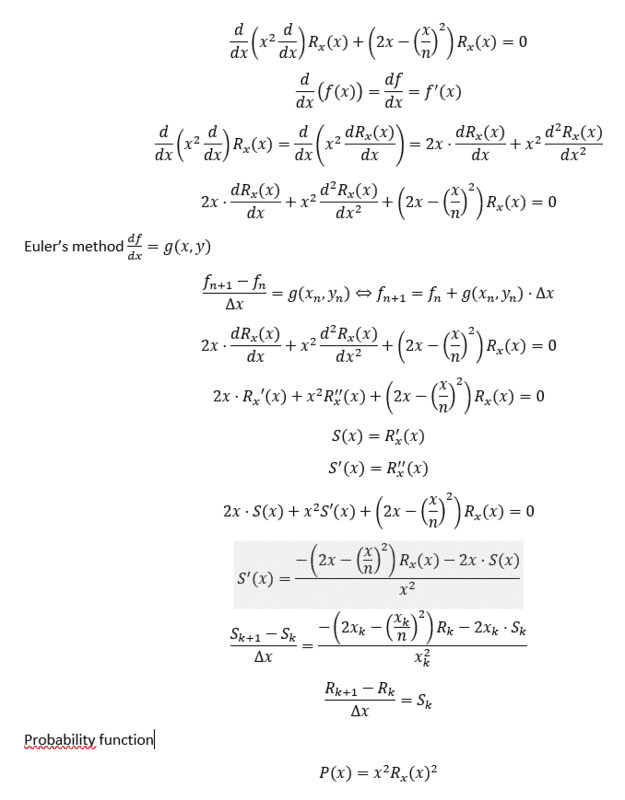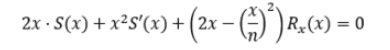# Solving Schrödinger's equation for a hydrogen atom with Euler's method

• I
• Tuca
In summary, @Tuca said that you need to know the energy eigenvalues beforehand or find the correct energy by trial and error (which is called "shooting method" and can utilize Euler's method or some other finite difference scheme). The correct eigenvalues make the wavefunction approach zero when ##r\rightarrow\infty##, as should be for physically possible states. @Hello said that you can use Euler's method only for spherically symmetric eigenstates of the H atom, and you also need to either know the energy eigenvalues beforehand or find the correct energy by trial and error (which is called "shooting method" and can utilize Euler's method or some other finite difference scheme

#### Tuca

Hi, first-time poster here

I'm a student at HS-level in DK, who has decided to write my annual large scale assignment on Schrödinger's equation. My teacher has only given us a brief introduction to the equation and has tasked us to solve it numerically with Euler's method for the hydrogen atom. He rewrote the equation on to a form I simply cannot seem to find online or anywhere else. My knowledge of using Euler's method for second-order ODEs is very sparse too. I have attached the equation. If someone can please help me understand, how I must use Euler's method on this specific form, I would be very thankful.

Thank youYou can use Euler's method only for spherically symmetric eigenstates of the H atom, and you also need to either know the energy eigenvalues beforehand or find the correct energy by trial and error (which is called "shooting method" and can utilize Euler's method or some other finite difference scheme). The correct eigenvalues make the wavefunction approach zero when ##r\rightarrow\infty##, as should be for physically possible states.

Hello @Tuca ,!​

I didn't reply to your post, since I don't have a ready answer. What I see in the picture seems to be the equation for the radial part of the wave function, suitably manipulated and simplified. I suppose there is no harm in peeking forward to the solutions, e.g. here . Picture e.g. https://users.aber.ac.uk/ruw/teach/327/hydrogen.php

You have a second order differential equation, so you need initial conditions.

Suppose you have ##R(0)## and ##S(0)## (and, for starters, ##n=1##) given, thengives you ##S'(0)## which, in combination with ##R'= S## allows you to take an Euler step.

I suppose you can start with ##R(0) = A = 1## and figure out a better value afterwards from the normalization requirement ##\int \text {probability} = 1##.

Now the weakness in my reply and the reason (*) it comes so late: I still don't know how to deal with ##S(0)## or some equivalent.

Maybe you or someone else has an idea ?

(*)Actually: one of the reasons. Another being that I tried to reproduce your radial equation from the separated Schrödinger equation -- and ended up with a mess.

Tuca said:
My knowledge of using Euler's method for second-order ODEs is very sparse too
Not much knowledge needed to upgrade from order 1 to two: Given ##f(0)## and ##f'(0)## you treat the latter as a 'new' function ##g##: ##g(x) = f'(x)## and then step both ##f## and ##g## :
##f_{n+1} = f_{n} + g_n \Delta x##​
##g_{n+1} = g_{n} + g'_n \Delta x##​
where g'_n follows from the second order equation.​

Hope this helps at least a little bit; keep us posted !

##\ ##